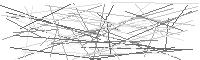# Applied Vocal Jury- Instructor Form

## Applied Vocal Jury- Instructor Form

• Student's Name Student's Name
• Class
Class
• ### Technique

• Must be a number less than or equal to 8.
• Must be a number less than or equal to 16.
• Must be a number less than or equal to 16.
• Must be a number less than or equal to 8.
• Must be a number less than or equal to 8.
• ### Artistry

• Must be a number less than or equal to 8.
• Must be a number less than or equal to 8.
• Must be a number less than or equal to 8.
• Must be a number less than or equal to 8.
• Must be a number less than or equal to 4.
• Must be a number less than or equal to 8.
•